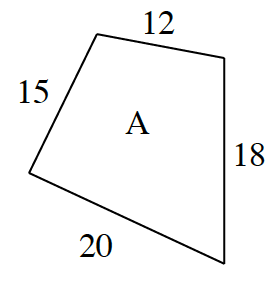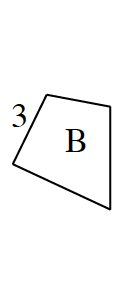### Home > CC2 > Chapter 4 > Lesson 4.3.2 > Problem4-94

4-94.

Figures A and B at right are similar. Assuming that figure A is the original figure, find the scale factor and find the lengths of the missing sides of figure B.Use the ratio between the known sides to find unknown sides.

What is the ratio between the two corresponding side lengths: $15$ and $3$?

New sides: $4$,$\frac{18}{5}$, and $\frac{12}{5}$

What would the scale factor be?